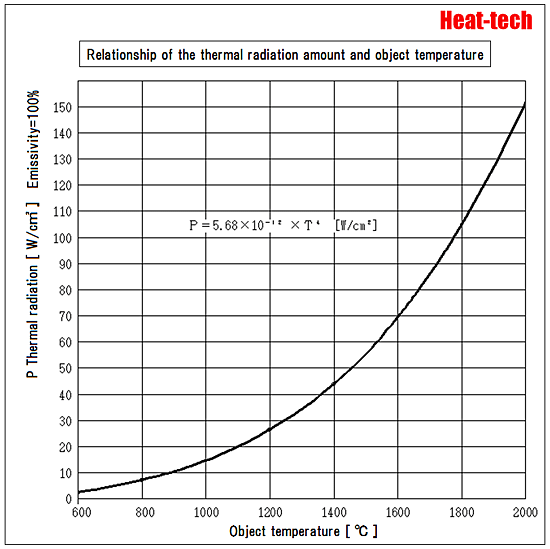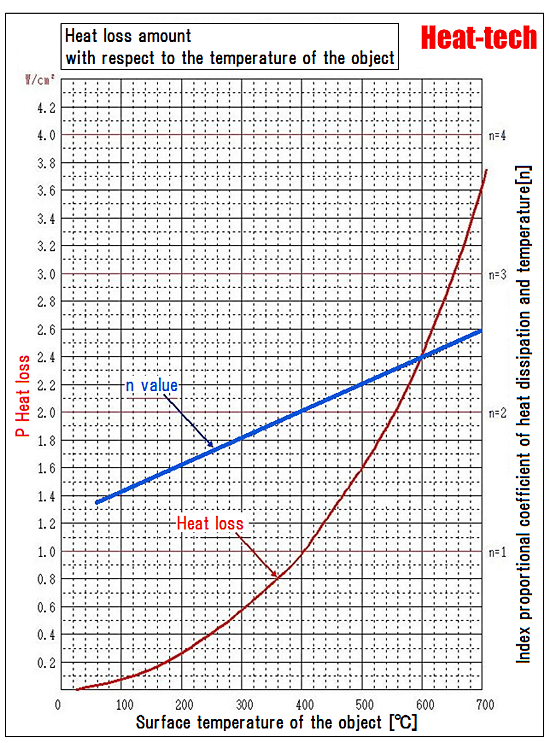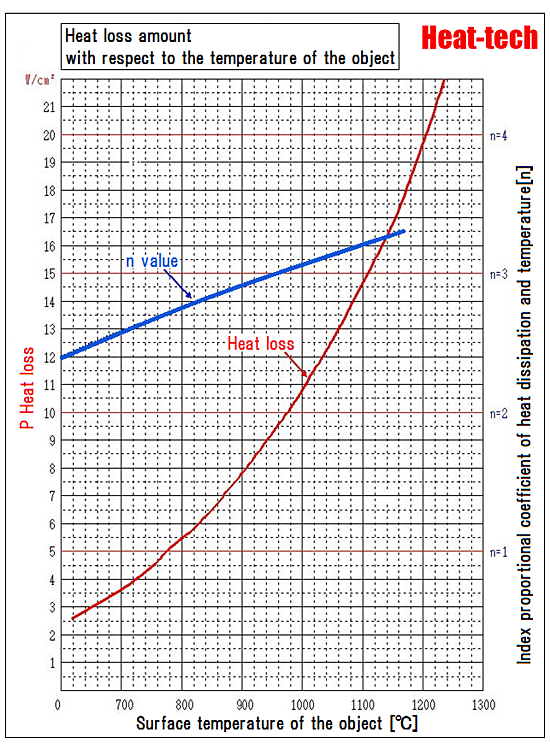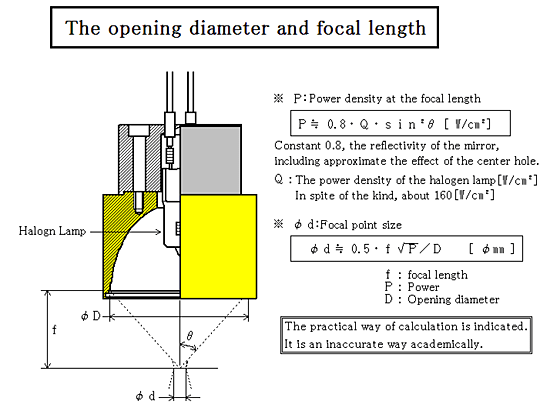Home / Halogen Point Heater / Basic knowledge of Halogen heater / 5.Physics of light heating / 5-2.About the temperature radiation intensity

It dissipates heat by radiation in the form of electromagnetic waves (~ microwave ~ infrared to visible light ~) when it comes to a temperature at which there is an object.
The proportion of thermal energy that is radiated from an object of high temperature, it becomes a high rate as high temperatures, this radiation will account for as many in the 500 ℃ or more than ambient.
Heat dissipation by conduction and convection is dominant in the 500 ℃ or less.

If the heat dissipation by radiation assumes an objective temperature to be T [K] and assumes emissivity to be 100%

Ｐ＝5.68×10-12 ×Ｔ4　[W/cm2] 　　(Stefan Boltzmann formula)Value shown above is a special situation of 100% emissivity at absolute zero degree ambient temperature.
In everyday life 300K ambient temperature is (27 ℃) around.

Therefore, those in the general form of equation Stefan Boltzmann as representative of the temperature radiated from an object when it is set to 0 [K] and the ambient temperature T is the following formula.

Ｐ＝5.68×10-12×(Ｔ4　-Ｔ04 )　　[W/cm2]

Definition is difficult to be exact, this expression is also approximate expression of that ambient temperature.

Know from this formula, the difference between the ambient temperature and the object temperature is zero, temperature radiation energy will also be zero.
The value of the expression is negative temperature of the object is lower than the ambient temperature.
This means that the thermal energy flows into the object.

Radiation formula by Stefan Boltzmann law of the first does not mean wrong.
Temperature radiation has come out from the body at a given temperature, radiation corresponding to the absolute temperature of about 300K even at room temperature has come out.
However as long as the ambient temperature is not absolute zero, I will receive the temperature radiation from the surroundings.
It becomes zero deduction ambient temperature is the same as the temperature of the object, and out of energy from the object is lost.

In addition, radiation is proportional to the fourth power of the temperature.
Temperature of an object is high enough compared to the ambient temperature, the temperature of the emission amount from the object is almost negligible the influence of the ambient temperature.
But the influence of the ambient temperature will be enormous differences in ambient temperature and body temperature becomes smaller.

Measured data of radiation amount with respect to the temperature of the object

The following is the actual measurement data of the amount of heat radiated from there, and the surface temperature of an object of the actual. (Room temperature 25 ℃)

The object has a dark appearance to one that is wound in close contact to be the outer diameter of φ23.5 long enough iron chromium aluminum wire of φ1, was fully oxidized.
Emissivity is estimated to be about 0.75 to 0.8, has an emissivity of almost the same oxide surface of stainless steel, it would be heat dissipation characteristics similar to the work of many of the actual.
However, it will be a low value is more heat dissipation in metal or glossy ceramic.

Heat loss amount with respect to the temperature of the object is represented by the following formula Broadly speaking in a narrow temperature range.

Ｐ≒ｋＴｎ
P: amount of heat loss [w / cm2] k: constant
T: Temperature of an object [℃] → The temperature difference δT with the surroundings exactly

The actually measured this relationship, was summarized is the following graph.
However, the index n varies with temperature as the blue curve is not constant.
Because heat loss by combining conduction, convection and radiation.
Conduction almost n = 1, convection almost n = 2, radiation almost n = 4.
Heat conduction is dominant at lower temperatures.
Heat dissipation is convection increases with temperature increases.
The proportion of radiation continue to increase rapidly from 200 ℃ – 300 ℃ per, this radiation becomes dominant at 500 ℃ or more.
The radiation will account for almost at several thousand ℃.
This means that the index n will approach 4 in the high-temperature range.Temperature measurement was used for the optical pyrometer (CHINO IR-U) is more than 700 ℃.
700 ℃ or less were used thermocouple φ0.32 but, thermocouple, so temperature is measured to be lower in general (because the measuring point is cooled in the thermocouple wire itself), the light at 700 ℃ or more compares the value of the pyrometer, we have to correct the measured value of the thermocouple in the low temperature range both the ratio of the measured temperature in there as can be applied even below 700 ℃.
This correction value was + 12%.
From this, it will be assumed to be measured to be lower by about 12% in the case of using a thermocouple on the surface φ0.32 temperature measurement of an object.
It must be aware of this point in the temperature of a thermocouple.
It could also put a thermocouple in the heating wire coil, but it appeared to be out the measured value is higher than the surface temperature of the reverse true in this case.Temperature of the object continue to rise, heat loss will continue to increase rapidly shown in the figure above.
To keep the temperature to the desired object, it must continue to provide the thermal energy of as much as the heat loss at that temperature.
For example, when heat the thin plate, which is held in the air, the heat dissipation area must be calculated in the front and back.
In the figure above, temperature radiation at 1400 ℃ ,it is about 44 [W / cm2], but it heat dissipation of about 88 [W / cm2] The addition of the back.
In other words, when heated to 1400 ℃ by the Halogen Point Heater, it need at least energy density 90 [W / cm2].
Then, if the absorption rate of 80%, it is necessary to heat with the light power density of 1100 [W / cm2].In the case of 0.5, F value, which is the point heater for elliptical mirror standard (f / D) power at the focal position

P ≒ 0.8 · Q · sin2 θ [W / cm2]
Q ≒ 160 [W / cm2]
Density is about 64 [W / cm2]